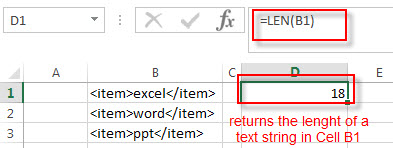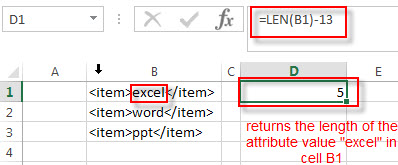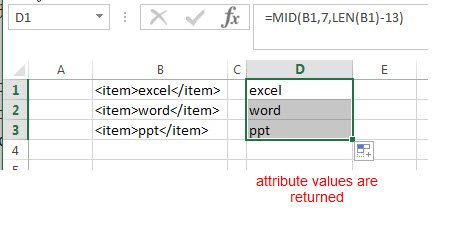# How to Extract Attribute Values from XML/HTML Data

This post will guide you how to extract attribute values from XML or HTML data in a range or cells in excel.  How to strip values from XML or other markup in excel Cells.

## Extract Attribute Values from XML

Assuming that you have a table that contains the content of the markup language, such as: XML or HTML markup data. If you want to extract only attribute values from xml data, you can use a combination of the MID function and the LEN function to create a new excel formula.

The format of markup value is like this:

```<item>excel</item>

<item>word</item>

<item>ppt</item>```

To strip attribute value in Cell B1, you can use the following formula:

`=MID(B1,7,LEN(B1)-13)`

Let’s see how this formula works:

=LEN(B1)The LEN function returns the length of the text string in Cell B1. It returns 18.

=LEN(B1)-13This formula returns the length of the attribute value in Cell B1, it use the length value of the cell B1 to subtract 13.  The number 13 is the length of the markup containing the starting tag (<item>) and the closing tag (</item>).

=MID(B1,7,LEN(B1)-13)The length of the starting tag is 6, so the position of the first character that you want to extract in attribute value in Cell B1 is 7.  And the num_chars is returned by the above LEN function as 5.  So the MID function extracts the attribute value between two markup tags as “excel”.

Note: you can drag the Fill Handle down to other cells to extract attribute values from other Cells (B2:B3).

### Related Formulas

• Remove Numeric Characters from a Cell
If you want to remove numeric characters from alphanumeric string, you can use the following complex array formula using a combination of the TEXTJOIN function, the MID function, the Row function, and the INDIRECT function..…
• Split Text String to an Array
If you want to convert a text string into an array that split each character in text as an element, you can use an excel formula to achieve this result. the below will guide you how to use a combination of the MID function, the ROW function, the INDIRECT function and the LEN function to split a string…
• remove non numeric characters from a cell
If you want to remove non numeric characters from a text cell in excel, you can use the array formula:{=TEXTJOIN(“”,TRUE,IFERROR(MID(B1,ROW(INDIRECT(“1:”&LEN(B1))),1)+0,””))}
• Get the position of Last Occurrence
If you want to get the position of the last occurrence of a character in a cell, then you can use a combination of the LOOKUP function, the MID function, the ROW function, the INDIRECT function and the LEN function to create an excel formula…

### Related Functions

• Excel MID function
The Excel MID function returns a substring from a text string at the position that you specify.The syntax of the MID function is as below:= MID (text, start_num, num_chars)…
• Excel LEN function
The Excel LEN function returns the length of a text string (the number of characters in a text string).The LEN function is a build-in function in Microsoft Excel and it is categorized as a Text Function.The syntax of the LEN function is as below:= LEN(text)…
Related Posts

Abbreviate Names Or Words in Excel

As an MS Excel user, you might have come across a task where you need to abbreviate different names or words, and there are also possibilities that you might have done this task manually by assuming that there isn't any ...

Extract Last Two Words From Multiple Cells

Just assume that you have a few cells containing values/words and you want to extract the last two words from each cell into another separate cell; then you might think that it's not a big deal; because you would prefer ...

Extract Multiple Lines From A Cell

Suppose that you have listed some text in a single cell which is separated by the line break(you can do it by pressing ALT + ENTER after entering the text), and now you want to extract multiple lines of text ...

Extract substring In Excel

This post will guide you how to use Excel's MID function is a quick and easy way to extract pieces from your text. Use the Excel formula to extract a substring with MID. Note: If you want to extract just ...

How to Count Numbers Nth Digit Equals to Specific Number in Excel

This post will guide you how to count numbers where the Nth digit equal to a specific number in Excel 2013/2016 or Excel office 365. How do I count of numbers in a given range where the Nth digit is ...

How to Convert Text to Time in Excel

This post will guide you how to convert time string to time in Excel. Assuming that you have a list of text string in your worksheet, and you wish to convert these time string to standard time format, how to ...

How to Extract Initials From a Name in Excel

This post will guide you how to get initials from a given name using a formula in Excel. How do I extract initials from names in Excel 2013/2016. Extract Initials from a Name Using a Formula Extract Initials from a ...

How to Extract Number from Text String in Excel

This post will guide you how to extract number from a given test string in Excel. How do I extract all numbers from string using a formula in Excel. How to get all number from a given test string using ...

How to Convert mmddyy to Date in Excel

This post will guide you how to convert non-standard date formats or text to a standard date in Excel. If you have a date with mmddyy format, and you want to change it as the normal date format as mm/dd/yyyy ...

How to Convert Military Time to Standard Time in Excel

This post will guide you how to convert military time to standard time with a formula in Excel. How do I convert from military time 17:29:30 to regular time 5:29:30PM in Excel.  How do I Convert Standard time to military ...

Sidebar